# Multiplexed mass cytometry profiling of cellular states perturbed by small-molecule regulators

Single cell analysis is a powerful method that allows for the deconvolution of the effect of treatments on complex populations containing different cell types, that may or may not respond to specific treatments. Depending on the technology used, the analytes can be genes, transcripts, proteins or metabolites. Using mass cytometry, bodenmiller et al measured the level of 9 proteins and 14 post-translational modifications. After using signal intensity from the 9 proteins (so called phenotypic markers) to define 14 sub-populations, they monitored the effect of several treatments using the 14 post-translational modifications.

Modeling and visualization of these type of data is challenging: the large number of events measured combined to the complexity of each samples is making the modelling complex, while the high dimensionality of the data precludes the use of standard visualizations.

The goal of this package is to enable the development of new methods by providing a curated set of data for testing and benchmarking.

# Data acquisition and preparation

For details on data acquisition please refer to Bodenmiller et al Nat Biotech 2012. Briefly, after treatment cells where profiled using a CyTOF, dead cells and debris were excluded and live cells were assigned to 1 of the 14 sub-populations using signal intensity from 9 phenotypic markers.

Samples corresponding to untreated cells, stimulated with BCR/FcR-XL, PMA/Ionomycin or vanadate or unstimulated, were downloaded from CytoBank as FCS files. Data was extracted and normalized using the arcsinh function with a cofactor of 5.

# The Reference dataset

The reference dataset corresponds to unstimulated, untreated samples. Data for the phenotypic markers can be visualized using a simple boxplot. First we turn the dataset into a Tall-Skinny data.frame

data(refPhenoMat)
refPhenoFrame <- melt(refPhenoMat)
names(refPhenoFrame) <- c('cell_id','channel','value')
ggplot(data=refPhenoFrame,aes(x=channel,y=value))+
geom_boxplot()+
theme(axis.text.x = element_text(angle = 45, hjust = 1))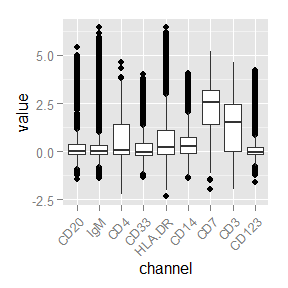We can also use boxplots to visualize the intensity of a single channel over all populations. We first load the cell annotations, add it to the phenotypic data, and define a single color per cell type:

data('refAnnots')
refPhenoFrame$Cells <- rep(refAnnots$Cells,ncol(refPhenoMat))
cell.colors <- setNames(c('#9CA5D5','#0015C5','#5B6CB4','#BFC5E8','#C79ED0','#850094',
'#A567B1','#DBBCE2','#D3C6A1','#5E4500','#BBDEB1','#8A1923',
'#B35E62','#CEA191'),
'cd4+','cd8+','dendritic','igm-','igm+','nk'))

And then

cd7.pops <- refPhenoFrame %>% filter(channel=='CD7')
ggplot(data=cd7.pops,
aes(x=Cells,y=value,fill=Cells))+
geom_boxplot()+
scale_fill_manual(values=cell.colors)+
guides(fill=FALSE)+
theme(axis.text.x = element_text(angle = 45, hjust = 1))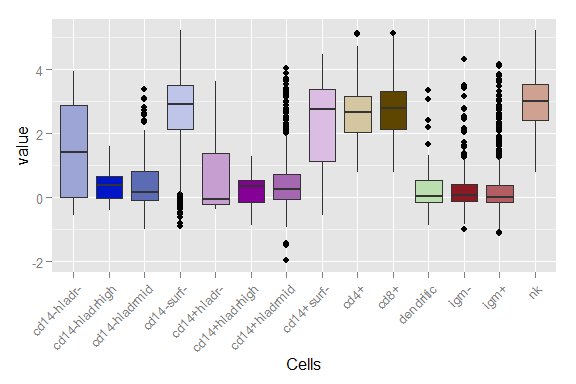Alternatively one can use fan plots to visualize the trends of values of all markers in a population:

ggplot(refPhenoFrame %>% filter(Cells=='cd4+') %>% group_by(Cells,channel) %>% do(do.fan(.$value)), aes(x=channel,fill=percent,group=id))+ geom_ribbon(aes(ymin=ymin,ymax=ymax))+ guides(fill=F)+ facet_wrap(~Cells)+ theme(axis.text.x = element_text(angle = 45, hjust = 1))+ scale_fill_fan()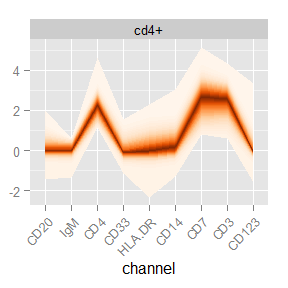For each population, the level of activation of the different pathways monitored using the functional markers can be visualized in the same way: data(refFuncMat) refFuncFrame <- melt(refFuncMat) names(refFuncFrame) <- c('cell_id','channel','value') refFuncFrame$Cells <- rep(refAnnots$Cells,ncol(refFuncMat)) ggplot(refFuncFrame %>% filter(Cells=='cd4+') %>% group_by(Cells,channel) %>% do(do.fan(.$value)),
aes(x=channel,fill=percent,group=id))+
geom_ribbon(aes(ymin=ymin,ymax=ymax))+
facet_wrap(~Cells)+
guides(fill=FALSE)+
theme(axis.text.x = element_text(angle = 45, hjust = 1))+
scale_fill_fan()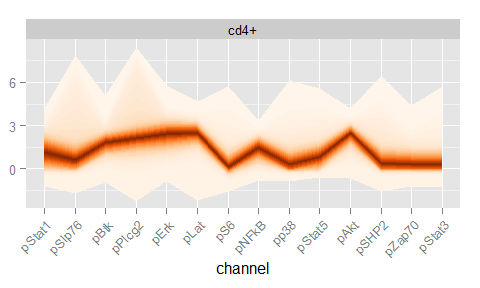# The Untreated dataset

The untreated dataset corresponds to unstimulated samples that have been treated with BCR/FcR-XL, PMA/Ionomycin or vanadate. To visualize the effect of the different treatments on cell populations, we first turn the dataset into a Tall-Skinny data.frame

data('untreatedFuncMat')
data('untreatedAnnots')
untreatedFuncFrame <- melt(untreatedFuncMat)
names(untreatedFuncFrame) <- c('cell_id','channel','value')
untreatedFuncFrame$Cells <- rep(untreatedAnnots$Cells,ncol(untreatedFuncMat))
untreatedFuncFrame$Treatment <- rep(untreatedAnnots$Treatment,ncol(untreatedFuncMat))

Then visualize the effects of the different stimulations on cd4+ cells:

refFuncLine <- refFuncFrame %>% filter(Cells=='cd4+') %>% group_by(Cells,channel) %>% summarise(value=median(value))
refFuncLine <- do.call(rbind,lapply(seq(1,3),function(x) refFuncLine))
refFuncLine$Treatment <- rep(levels(untreatedFuncFrame$Treatment),each=nlevels(refFuncLine$channel)) refFuncLine$percent <- 0
refFuncLine$id <- 'ref' ggplot(untreatedFuncFrame %>% filter(Cells=='cd4+') %>% group_by(Treatment,Cells,channel) %>% do(do.fan(.$value)),
aes(x=channel,fill=percent,group=id))+
geom_ribbon(aes(ymin=ymin,ymax=ymax))+
geom_line(data=refFuncLine,aes(y=value),
col='black',linetype=4)+
guides(fill=F)+
facet_wrap(~Cells*Treatment,ncol=2,scale='free_x')+
theme(axis.text.x = element_text(angle = 45, hjust = 1))+
scale_fill_fan()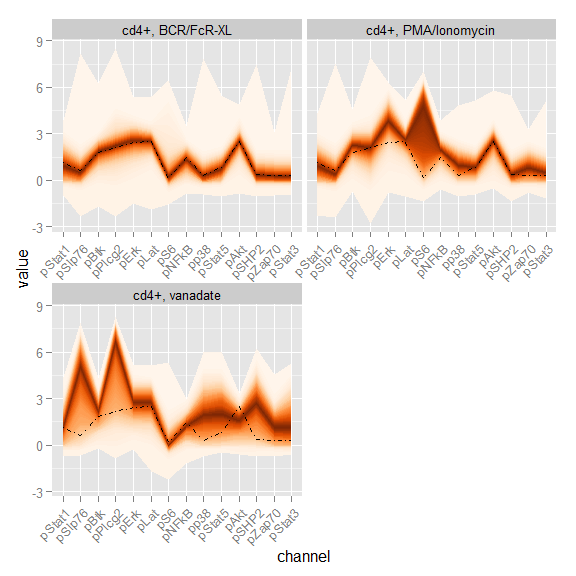The dotted line corresponds to the median value of the reference sample for each channel.